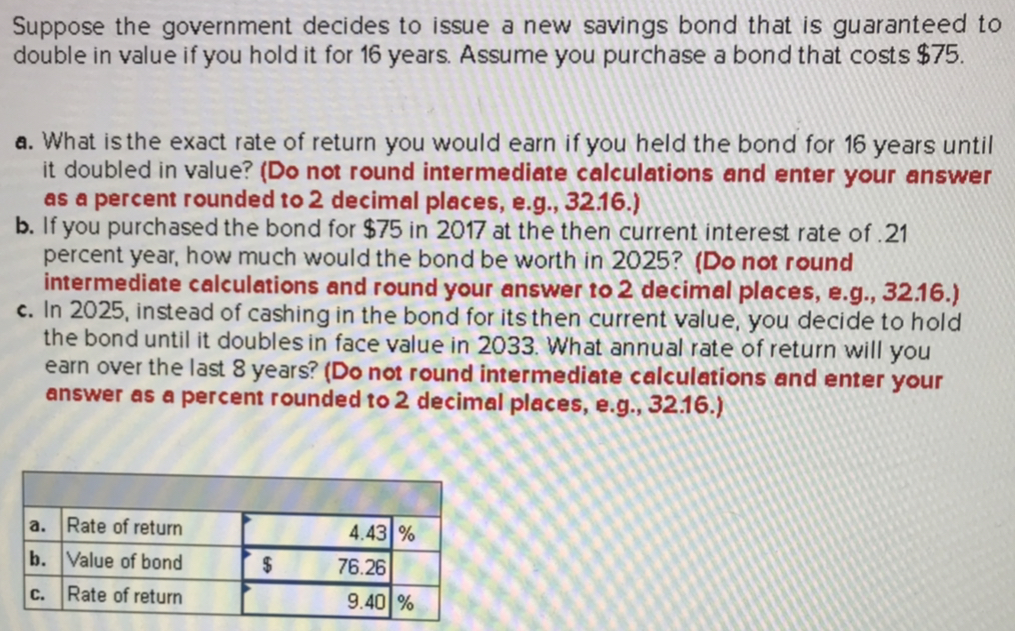Suppose the government decides to issue a new savings bond that is guaranteed todouble in value if you hold it for 16 years. Assume you purchase a bond that costs \$75.a. What is the exact rate of return you would earn if you held the bond for 16 years untilb. If you purchased the bond for \$75 in 2017 at the then current interest rate of.21c. In 2025, instead of cashing in the bond for its then current value, you decide to holdit doubled in value? (Do not round intermediate calculations and enter your answeras a percent rounded to 2 decimal places, e.g., 32.16.)percent year, how much would the bond be worth in 2025? (Do not roundintermediate calculations and round your answer to 2 decimal places, e.g., 32.16.)the bond until it doubles in face value in 2033. What annual rate of return will youearn over the last 8 years? (Do not round intermediate calculations and enter youranswer as a percent rounded to 2 decimal places,e.g..32.16.)a. Rate of returnb. Value of bondc. Rate of return4.43%76.269.4 %

Questionhelp_outlineImage TranscriptioncloseSuppose the government decides to issue a new savings bond that is guaranteed to double in value if you hold it for 16 years. Assume you purchase a bond that costs \$75. a. What is the exact rate of return you would earn if you held the bond for 16 years until b. If you purchased the bond for \$75 in 2017 at the then current interest rate of.21 c. In 2025, instead of cashing in the bond for its then current value, you decide to hold it doubled in value? (Do not round intermediate calculations and enter your answer as a percent rounded to 2 decimal places, e.g., 32.16.) percent year, how much would the bond be worth in 2025? (Do not round intermediate calculations and round your answer to 2 decimal places, e.g., 32.16.) the bond until it doubles in face value in 2033. What annual rate of return will you earn over the last 8 years? (Do not round intermediate calculations and enter your answer as a percent rounded to 2 decimal places,e.g..32.16.) a. Rate of return b. Value of bond c. Rate of return 4.43% 76.26 9.4 % fullscreen
Step 1

Step1: Calculating the value of rate of return on the investment in the bond. We have,

The relationship between present value and future value of money is explained by the following equation:

Future value = Present Value (1+r)n

Here,

Present Value = \$ 75

Future value = \$ 150

Number of years (n) = 16 years

Rate of return(r) =?

By substituting these value in the above formula. We get;

\$ 150 = \$ 75 (1+r)16

(1+r)16 = \$ 150 / \$ 75

(1+r)16 = 2

(1+r) = (2)1/16

(1 + r) = 1.0443

r = 1.0443 – 1

r = 0.0443 x 100

r= 4.43 %

Therefore the value of rate of return on the investment in the bond shall be 4.43%.

Step 2

Step2: Calculating the future value of the bond in the year 2025. We have,

The relationship between present value and future value of money is explained by the following equation:

Future value = Present Value (1+r)n

Here,

Present Value = \$ 75

Future value =?

Number of years (n) = 8 years

Rate of return(r) = 0.21%

By substituting these ...

Want to see the full answer?

See Solution

Want to see this answer and more?

Our solutions are written by experts, many with advanced degrees, and available 24/7

See Solution
Tagged in